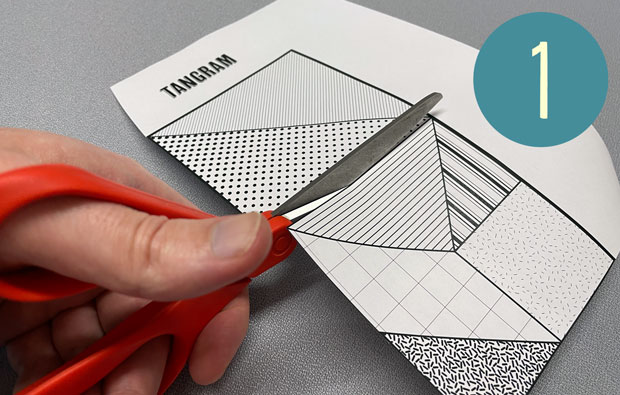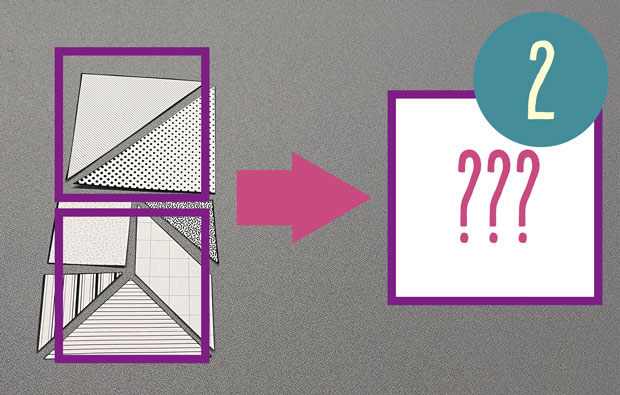# What's new## Try a tangram

, 20 July 2022See if you can solve this ancient shape puzzle

This traditional Chinese puzzle, known as a tangram, reveals some surprising properties of squares!

### You will need

• A printed copy of the tangram template [PDF, 541 kB]
• Scissors
• Pens or pencils for colouring in (optional)

### What to do

1.Cut out each of the pieces. Arrange the pieces on a surface so their positions match the arrangement in the printout.
2.The pieces make two squares that are the same size.  Can you take all the pieces and put them together to make one large square?

### What’s happening?

After taking two smaller squares and turning them into one, the new square will be bigger. That means its sides must be longer, too. But how much longer?

When you finish the puzzle, you’ll find that the largest triangles have their diagonals along the edges of the square. Those sides were originally the diagonal of one of the smaller squares.

The new sides are longer than the sides of the smaller triangles. If they were twice as long, you’d be able to fit four smaller squares inside the big square – one in each corner. Clearly that’s too big, so the new sides are less than twice as long.

### Real-life maths

The diagonal of a square is connected to a number known as the square root of two. This can also be written with the symbol √2. A 1-metre square has a diagonal that is √2 metres long.

The √2 has a special property. When you multiply √2 by itself, you get 2. If you had a giant tangram puzzle that started as a pair of 1-metre squares, the solution would have sides that were √2 metres long. If you calculate the area of that square, it’s √2 x √2, which equals 2 square metres!

In some ways, √2 is a tricky number to write down. If you write it as a decimal, it starts out as 1.4142… but it keeps going forever. Even stranger, the decimal for √2 will never get stuck in a loop like the decimal for 1/3 does (1/3 = 0.33333…).

If you want to find the length of the diagonal for any square, multiply the side length by √2. This is a useful measurement for builders, and mathematicians have been working out values for √2 for thousands of years. The ancient Babylonians worked out a value for √2 that was accurate to six decimal places in 1600 BCE!

Categories: